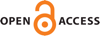Download this articleFor screen For printingRecent IssuesThe Journal About the Journal Editorial Board Editors’ Interests Subscriptions Submission Guidelines Submission Form Policies for Authors Ethics Statement ISSN: 1944-7833 (e-only) ISSN: 1937-0652 (print) Author Index To Appear Other MSP JournalsOn unipotent radicals of motivic Galois groups

### Payman Eskandari and V. Kumar Murty

Vol. 17 (2023), No. 1, 165–215##### Abstract

Let $T$ be a neutral Tannakian category over a field of characteristic zero with unit object $⊮$, and equipped with a filtration ${W}_{\bullet }$ similar to the weight filtration on mixed motives. Let $M$ be an object of $T$, and $\underset{¯}{\mathfrak{𝔲}}\left(M\right)\subset {W}_{-1}\underset{¯}{\mathrm{Hom}}\left(M,M\right)$ the Lie algebra of the kernel of the natural surjection from the fundamental group of $M$ to the fundamental group of ${\mathrm{Gr}}^{W}M$. A result of Deligne gives a characterization of $\underset{¯}{\mathfrak{𝔲}}\left(M\right)$ in terms of the extensions $0\to {W}_{p}M\to M\to M∕{W}_{p}M\to 0$: it states that $\underset{¯}{\mathfrak{𝔲}}\left(M\right)$ is the smallest subobject of ${W}_{-1}\underset{¯}{\mathrm{Hom}}\left(M,M\right)$ such that the sum of the aforementioned extensions, considered as extensions of $⊮$ by ${W}_{-1}\underset{¯}{\mathrm{Hom}}\left(M,M\right)$, is the pushforward of an extension of $⊮$ by $\underset{¯}{\mathfrak{𝔲}}\left(M\right)$. We study each of the above-mentioned extensions individually in relation to $\underset{¯}{\mathfrak{𝔲}}\left(M\right)$. Among other things, we obtain a refinement of Deligne’s result, where we give a sufficient condition for when an individual extension $0\to {W}_{p}M\to M\to M∕{W}_{p}M\to 0$ is the pushforward of an extension of $⊮$ by $\underset{¯}{\mathfrak{𝔲}}\left(M\right)$. In the second half of the paper, we give an application to mixed motives whose unipotent radical of the motivic Galois group is as large as possible (i.e., with $\underset{¯}{\mathfrak{𝔲}}\left(M\right)={W}_{-1}\underset{¯}{\mathrm{Hom}}\left(M,M\right)$). Using Grothendieck’s formalism of extensions panachées we prove a classification result for such motives. Specializing to the category of mixed Tate motives we obtain a classification result for 3-dimensional mixed Tate motives over $ℚ$ with three weights and large unipotent  radicals.

##### Keywords
mixed motives, motivic Galois groups, periods
##### Mathematical Subject Classification
Primary: 14F42
Secondary: 11M32, 18M25, 32G20
##### Milestones